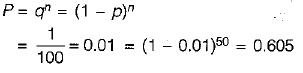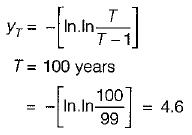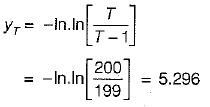Courses

# Test: Floods - 1

## 10 Questions MCQ Test GATE Civil Engineering (CE) 2022 Mock Test Series | Test: Floods - 1

Description
This mock test of Test: Floods - 1 for Civil Engineering (CE) helps you for every Civil Engineering (CE) entrance exam. This contains 10 Multiple Choice Questions for Civil Engineering (CE) Test: Floods - 1 (mcq) to study with solutions a complete question bank. The solved questions answers in this Test: Floods - 1 quiz give you a good mix of easy questions and tough questions. Civil Engineering (CE) students definitely take this Test: Floods - 1 exercise for a better result in the exam. You can find other Test: Floods - 1 extra questions, long questions & short questions for Civil Engineering (CE) on EduRev as well by searching above.
QUESTION: 1

### Of the various empirical formulae relating the flood peak to the catchment area,

Solution:

Dickens formula is used in the Central and Northern parts of the country. Ryves formula is used in Tamil Nadu and parts of Karnataka and Andhra Pradesh. Inglish formula is used in Western Ghats in Maharashtra.

QUESTION: 2

Solution:
QUESTION: 3

### The probability that a hundred year flood may not occur at all during the 50 year life of the project is

Solution:QUESTION: 4

The general equation for hydrological frequency analysis states that xT = value of a variate with a return period of T years is given by xT

Solution:
QUESTION: 5

For a return period of 100 years the Gumbel’s reduced variate yT is

Solution:QUESTION: 6

An annual flood series contains 100 years of flood data. For a return period of 200 years the Gumbel’s reduced variate is

Solution:

Gumbel’s reduced variate, yT,QUESTION: 7

To use Gumbel’s method to estimate the magnitude of a flood with a return period of T years, the following data pertaining to annual flood series are sufficient

Solution:
QUESTION: 8

To estimate the flood magnitude with a return period T by the log-Pearson Type III method, the following data pertaining to annual flood series are sufficient

Solution:
QUESTION: 9

To estimate the flood magnitude with a return period of T years by using log-normal distribution, the following statistics of data are absolutely necessary

Solution:
QUESTION: 10

The recurrence interval of an event is TA in annual series and Tp in partial-duration series, then

Solution: